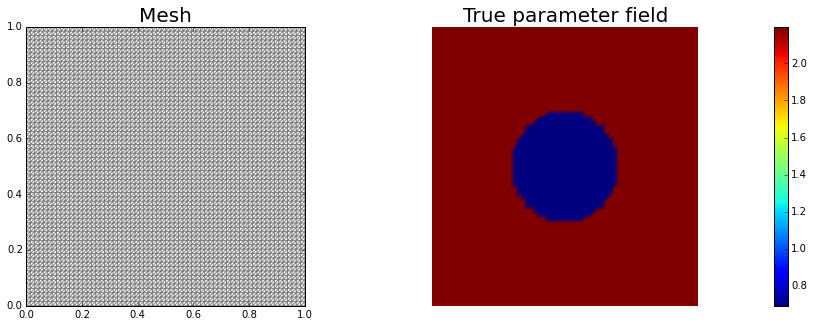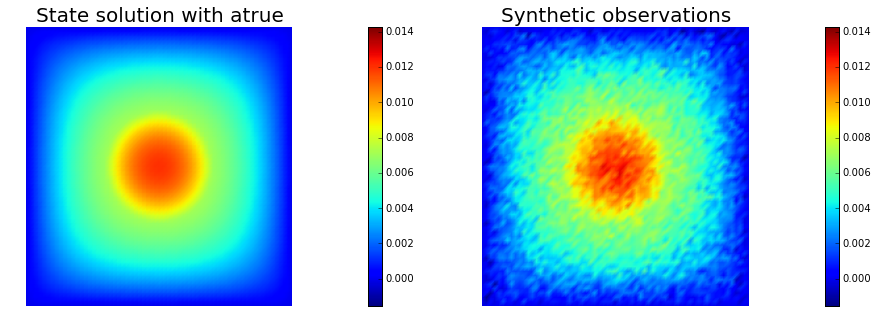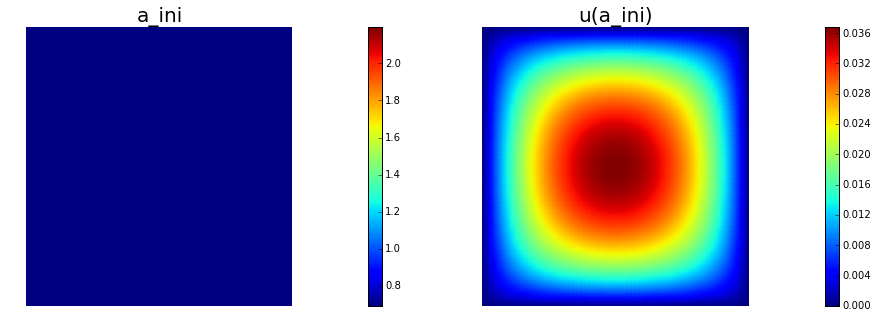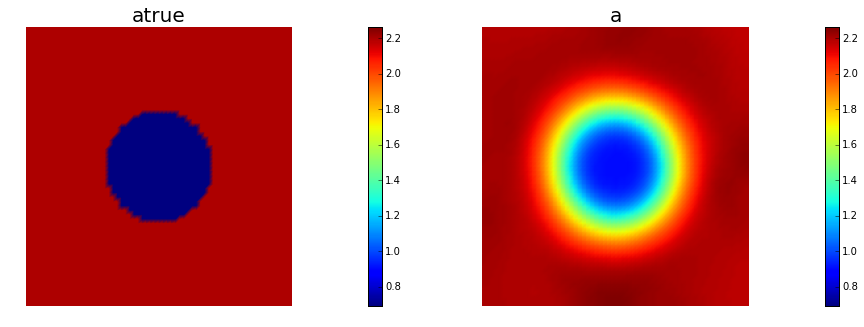# Example: Coefficient field inversion in an elliptic partial differential equation¶

We consider the estimation of a coefficient in an elliptic partial differential equation as a first model problem. Depending on the interpretation of the unknowns and the type of measurements, this model problem arises, for instance, in inversion for groundwater flow or heat conductivity. It can also be interpreted as finding a membrane with a certain spatially varying stiffness. Let $\Omega\subset\mathbb{R}^n$, $n\in\{1,2,3\}$ be an open, bounded domain and consider the following problem:

$$\min_{a} J(a):=\frac{1}{2}\int_\Omega (u-u_d)^2\, dx + \frac{\gamma}{2}\int_\Omega|\nabla a|^2\,dx,$$

where $u$ is the solution of

$$\begin{split} \quad -\nabla\cdot(\exp(a)\nabla u) &= f \text{ in }\Omega,\\ u &= 0 \text{ on }\partial\Omega. \end{split}$$

Here $a\in U_{ad}:=\{a\in L^{\infty}(\Omega)\}$ the unknown coefficient field, $u_d$ denotes (possibly noisy) data, $f\in H^{-1}(\Omega)$ a given force, and $\gamma\ge 0$ the regularization parameter.

### The variational (or weak) form of the state equation:¶

Find $u\in H_0^1(\Omega)$ such that $(\exp(a)\nabla u,\nabla v) - (f,v) = 0, \text{ for all } v\in H_0^1(\Omega),$ where $H_0^1(\Omega)$ is the space of functions vanishing on $\partial\Omega$ with square integrable derivatives. Here, $(\cdot\,,\cdot)$ denotes the $L^2$-inner product, i.e, for scalar functions $u,v$ defined on $\Omega$ we denote $(u,v) := \int_\Omega u(x) v(x) \,dx$.

### Optimality System:¶

The Lagrangian functional $\mathscr{L}:L^\infty(\Omega)\times H_0^1(\Omega)\times H_0^1(\Omega)\rightarrow \mathbb{R}$, which we use as a tool to derive the optimality system, is given by

$$\mathscr{L}(a,u,p):= \frac{1}{2}(u-u_d,u-u_d) + \frac{\gamma}{2}(\nabla a, \nabla a) + (\exp(a)\nabla u,\nabla p) - (f,p).$$

The Lagrange multiplier theory shows that, at a solution all variations of the Lagrangian functional with respect to all variables must vanish. These variations of $\mathscr{L}$ with respect to $(p,u,a)$ in directions $(\tilde{u}, \tilde{p}, \tilde{a})$ are given by

\begin{alignat}{2} \mathscr{L}_p(a,u,p)(\tilde{p}) &= (\exp(a)\nabla u, \nabla \tilde{p}) - (f,\tilde{p}) &&= 0,\\ \mathscr{L}_u(a,u,p)(\tilde{u}) &= (\exp(a)\nabla p, \nabla \tilde{u}) + (u-u_d,\tilde{u}) && = 0,\\ \mathscr{L}_a(a,u,p)(\tilde{a}) &= \gamma(\nabla a, \nabla \tilde{a}) + (\tilde{a}\exp(a)\nabla u, \nabla p) &&= 0, \end{alignat}

where the variations $(\tilde{u}, \tilde{p}, \tilde{a})$ are taken from the same spaces as $(u,p,a)$.

The gradient of the cost functional $\mathcal{J}(a)$ therefore is

$$\mathcal{G}(a)(\tilde a) = \gamma(\nabla a, \nabla \tilde{a}) + (\tilde{a}\exp(a)\nabla u, \nabla \tilde{p}).$$

### Inexact Newton-CG:¶

Newton's method requires second-order variational derivatives of the Lagrangian . Written in abstract form, it computes an update direction $(\hat a_k, \hat u_k,\hat p_k)$ from the following Newton step for the Lagrangian functional:

$$\mathscr{L}''(a_k, u_k, p_k)\left[(\tilde a, \tilde u, \tilde p),(\hat a_k, \hat u_k, \hat p_k)\right] = -\mathscr{L}'(a_k,u_k,p_k)(\tilde a, \tilde u, \tilde p),$$

for all variations $(\tilde a, \tilde u, \tilde p)$, where $\mathscr{L}'$ and $\mathscr{L}''$ denote the first and second variations of the Lagrangian. For the elliptic parameter inversion problem, this Newton step (written in variatonal form) is as follows: Find $(\hat u_k, \hat a_k,\hat p_k)$ as the solution of the linear system

$$\begin{array}{llll} (\hat{u}_k, \tilde u) &+ (\hat{a}_k \exp(a_k)\nabla p_k, \nabla \tilde u) &+ (\exp(a_k) \nabla \tilde u, \nabla \hat p_k) &= (u_d - u_k, \tilde u)- (\exp(a_k) \nabla p_k, \nabla \tilde u)\\ (\tilde a \exp(a_k) \nabla \hat u_k, \nabla p_k) &+ \gamma (\nabla \hat a_k, \nabla \tilde a) + (\tilde a \hat a_k \exp(a_k)\nabla u, \nabla p) &+ (\tilde a \exp(a_k) \nabla u_k, \nabla \hat p_k) &= - \gamma (\nabla a_k, \nabla\tilde a) - (\tilde a \exp(a_k) \nabla u_k, \nabla p_k)\\ (\exp(a_k) \nabla \hat u_k, \nabla \tilde p) &+ (\hat a_k \exp(a_k) \nabla u_k, \nabla \tilde p) & &= - (\exp(a_k) \nabla u_k, \nabla \tilde p) + (f, \tilde p), \end{array}$$

for all $(\tilde u, \tilde a, \tilde p)$.

### Discrete Newton system:¶

$\def\tu{\tilde u} \def\btu{\bf \tilde u} \def\ta{\tilde a} \def\bta{\bf \tilde a} \def\tp{\tilde p} \def\btp{\bf \tilde p} \def\hu{\hat u} \def\bhu{\bf \hat u} \def\ha{\hat a} \def\bha{\bf \hat a} \def\hp{\hat p} \def\bhp{\bf \hat p}$ The discretized Newton step: denote the vectors corresponding to the discretization of the functions $\ha_k,\hu_k, \hp_k$ by $\bf \bha_k, \bhu_k$ and $\bhp_k$. Then, the discretization of the above system is given by the following symmetric linear system:

$$\begin{bmatrix} \bf W_{\scriptsize\mbox{uu}} & \bf W_{\scriptsize\mbox{ua}} & \bf A^T \\ \bf W_{\scriptsize\mbox{au}} & \bf R + \bf R_{\scriptsize\mbox{aa}}& \bf C^T \\ \bf A & \bf C & 0 \end{bmatrix} \left[ \begin{array}{c} \bhu_k \\ \bha_k \\ \bhp_k \end{array} \right] = -\left[ \begin{array}{ccc} \bf{g}_u\\ \bf{g}_a\\ \bf{g}_p \end{array} \right],$$

where $\bf W_{\scriptsize \mbox{uu}}$, $\bf W_{\scriptsize\mbox{ua}}$, $\bf W_{\scriptsize\mbox{au}}$, and $\bf R$ are the components of the Hessian matrix of the Lagrangian, $\bf A$ and $\bf C$ are the Jacobian of the state equation with respect to the state and the control variables, respectively and $\bf g_u$, $\bf g_a$, and $\bf g_p$ are the discrete gradients of the Lagrangian with respect to $\bf u$, $\bf a$ and $\bf p$, respectively.

### Reduced Hessian apply:¶

To eliminate the incremental state and adjoint variables, $\bhu_k$ and $\bhp_k$, from the first and last equations we use

\begin{align} \bhu_k &= -\bf A^{-1} \bf C \, \bha_k,\\ \bhp_k &= -\bf A^{-T} (\bf W_{\scriptsize\mbox{uu}} \bhu_k + \bf W_{\scriptsize\mbox{ua}}\,\bha_k). \end{align}

This results in the following reduced linear system for the Newton step

$$\bf H \, \bha_k = -\bf{g}_a,$$

with the reduced Hessian $\bf H$ applied to a vector $\bha$ given by

$$\bf H \bha = \underbrace{(\bf R + \bf R_{\scriptsize\mbox{aa}})}_{\text{Hessian of the regularization}} \bha + \underbrace{(\bf C^{T}\bf A^{-T} (\bf W_{\scriptsize\mbox{uu}} \bf A^{-1} \bf C - \bf W_{\scriptsize\mbox{ua}}) - \bf W_{\scriptsize\mbox{au}} \bf A^{-1} \bf C)}_{\text{Hessian of the data misfit}}\;\bha.$$

### Goals:¶

By the end of this notebook, you should be able to:

• solve the forward and adjoint Poisson equations
• understand the inverse method framework
• visualise and understand the results
• modify the problem and code

### Mathematical tools used:¶

• Finite element method
• inexact Newton-CG
• Armijo line search

### List of software used:¶

• FEniCS, a parallel finite element element library for the discretization of partial differential equations
• PETSc, for scalable and efficient linear algebra operations and solvers
• Matplotlib, a python package used for plotting the results
• Numpy, a python package for linear algebra

## Set up¶

### Import dependencies¶

In :
from dolfin import *

import numpy as np
import time
import logging

import matplotlib.pyplot as plt
%matplotlib inline
import nb

start = time.clock()

logging.getLogger('FFC').setLevel(logging.WARNING)
logging.getLogger('UFL').setLevel(logging.WARNING)
set_log_active(False)

np.random.seed(seed=1)


### The cost function evaluation:¶

$$J(a):=\underbrace{\frac{1}{2}\int_\Omega (u-u_d)^2\, dx}_{\text misfit} + \underbrace{\frac{\gamma}{2}\int_\Omega|\nabla a|^2\,dx}_{\text reg}$$
In :
# Define cost function
def cost(u, ud, a, W, R):
diff = u.vector() - ud.vector()
reg = 0.5 * a.vector().inner(R*a.vector() )
misfit = 0.5 * diff.inner(W * diff)
return [reg + misfit, misfit, reg]


### The reduced Hessian apply to a vector v:¶

\begin{align} \bhu &= -\bf A^{-1} \bf C \, & \text{linearized forward}\\ \bhp &= -\bf A^{-T} (\bf W_{\scriptsize\mbox{uu}} \bhu + \bf W_{\scriptsize\mbox{ua}}\,\bha) & \text{adjoint}\\ \bf H \bf v &= (\bf R + \bf R_{\scriptsize\mbox{aa}})\bf v + \bf C^T \bhp + \bf W_{\scriptsize\mbox{au}} \bhu. \end{align}
In :
# define (Gauss-Newton) Hessian apply H * v
def Hess_GN (v, R, C, A, W):
solve (A, du, - (C * v))
solve (A, dp, - (W * du))
CT_dp = Vector()
C.init_vector(CT_dp, 1)
C.transpmult(dp, CT_dp)
H_V = R * v + CT_dp
return H_V

# define (Newton) Hessian apply H * v
def Hess_Newton (v, R, Raa, C, A, W, Wua):
RHS = -(C * v)
bc2.apply(RHS)
solve (A, du, RHS)
RHS = -(W * du) -  Wua * v
bc2.apply(RHS)
solve (A, dp, RHS)
CT_dp = Vector()
C.init_vector(CT_dp, 1)
C.transpmult(dp, CT_dp)
Wua_du = Vector()
Wua.init_vector(Wua_du, 1)
Wua.transpmult(du, Wua_du)
H_V = R*v + Raa*v + CT_dp + Wua_du
return H_V

# Creat Class MyLinearOperator to perform Hessian function
class MyLinearOperator(LinearOperator):
cgiter = 0
def __init__(self, R, Raa, C, A, W, Wua):
LinearOperator.__init__(self, a_delta, a_delta)
self.R = R
self.Raa = Raa
self.C = C
self.A = A
self.W = W
self.Wua = Wua

# Hessian performed on x, output as generic vector y
def mult(self, x, y):
self.cgiter += 1
if iter <= 500:
y.set_local(Hess_GN (x, self.R, self.C, self.A, self.W).array() )
else:
y.set_local(Hess_Newton (x, self.R, self.Raa, self.C, self.A, self.W, self.Wua).array() )


### Model set up:¶

As in the introduction, the first thing we need to do is set up the numerical model. In this cell, we set the mesh, the finite element functions $u, p, g$ corresponding to state, adjoint and coefficient/gradient variables, and the corresponding test functions and the parameters for the optimization.

In :
# create mesh and define function spaces
nx = 64
ny = 64
mesh = UnitSquareMesh(nx, ny)
V = FunctionSpace(mesh, 'Lagrange', 1)
V2 = FunctionSpace(mesh, 'Lagrange', 2)

# define Trial and Test Functions
u, p, g, a_delta = TrialFunction(V2), TrialFunction(V2), TrialFunction(V), TrialFunction(V)
u_test, p_test, ud_test, g_test = TestFunction(V2), TestFunction(V2), TestFunction(V2), TestFunction(V)
p = Function(V2)

# initialize input functions
atrue = Expression('log(2 + 7*(pow(pow(x - 0.5,2) + pow(x - 0.5,2),0.5) > 0.2))')
f = Constant("1.0")
u0 = Constant("0.0")
a = interpolate(Expression("log(2.0)"),V)

# plot
atruef = interpolate(atrue, V)
plt.figure(figsize=(15,5))
nb.plot(mesh,subplot_loc=121, mytitle="Mesh", show_axis='on')
nb.plot(atruef,subplot_loc=122, mytitle="True parameter field")
plt.show()

# noise level
noise_level = 0.05

# define parameters for the optimization
tol = 1e-8
gamma = 1e-8
c = 1e-4
maxiter = 100
#eps = 1e-4
plot_on = 0

# initialize iter counters
iter = 1
total_cg_iter = 0
solution = 0In :
# set up dirichlet boundary conditions
def u0_boundary(x,on_boundary):
return on_boundary
bc2 = DirichletBC(V2, u0, u0_boundary)


### Set up synthetic observations:¶

• Propose a coefficient field $a_{\text true}$ shown above
• The weak form of the pde: Find $u\in H_0^1(\Omega)$ such that $\underbrace{(\exp(a_{\text true})\nabla u,\nabla v)}_{\; := \; a_{pde}} - \underbrace{(f,v)}_{\; := \;L_{pde}} = 0, \text{ for all } v\in H_0^1(\Omega)$.

• Perturb the solution: $u = u + \eta$, where $\eta \sim \mathcal{N}(0, \sigma)$

In :
# weak form for setting up the synthetic observations
ud = TrialFunction(V2)
L_goal = f * ud_test * dx

# solve the forward/state problem to generate synthetic observations
goal_A, goal_b = assemble_system(a_goal, L_goal, bc2)
ud = Function(V2)
solve(goal_A, ud.vector(), goal_b)

utrue = Function(V2)
utrue.assign(ud)

# perturb state solution and create synthetic measurements ud
# ud = u + ||u||/SNR * random.normal
MAX = ud.vector().norm("linf")
noise = Vector()
goal_A.init_vector(noise,1)
noise.set_local( noise_level * MAX * np.random.normal(0, 1, len(ud.vector().array())) )
ud.vector().axpy(1., noise)
bc2.apply(ud.vector())

# plot
nb.multi1_plot([utrue, ud], ["State solution with atrue", "Synthetic observations"])
plt.show()## Setting up the state equations, right hand side for the adjoint and the neccessary matrices:¶

In :
# weak form for setting up the state equation
L_state = f * u_test * dx
W_equ   = inner(u, u_test) * dx

# # weak form for setting up the right hand side of the adjoint
u = Function(V2)
L_adjoint = -inner(u - ud, u_test) * dx

# weak form for setting up matrices
M_equ   = inner(g, g_test) * dx
Raa_equ = inner(exp(a) * a_delta * g_test *  nabla_grad(u),  nabla_grad(p)) * dx

# assemble matrices M, W, and R
M = assemble(M_equ)
W = assemble(W_equ)
R = assemble(R_equ)

In :
# solve state equation
A, state_b = assemble_system (a_state, L_state, bc2)
solve (A, u.vector(), state_b)

# evaluate cost
[cost_old, misfit_old, reg_old] = cost(u, ud, a, W, R)

# plot
plt.figure(figsize=(15,5))
nb.plot(a,subplot_loc=121, mytitle="a_ini", vmin=atruef.vector().min(), vmax=atruef.vector().max())
nb.plot(u,subplot_loc=122, mytitle="u(a_ini)")
plt.show()## The inexact Newton-CG optimization with Armijo line search:¶

In :
# initializations
g, a_delta = Vector(), Vector()
R.init_vector(a_delta,0)
R.init_vector(g,0)

du, dp = Vector(), Vector()
W.init_vector(du,1)
W.init_vector(dp,0)

a_prev, a_diff = Function(V), Function(V)

print "Nit   CGit   cost          misfit        reg           sqrt(-G*D)    ||grad||       alpha  tolcg"

while iter <  maxiter and solution == 0:

# assemble matrix C
C =  assemble(C_equ)

# assemble W_ua and R
Wua = assemble (Wua_equ)
Raa = assemble (Raa_equ)

CT_p = Vector()
C.init_vector(CT_p,1)
C.transpmult(p.vector(), CT_p)
MG = CT_p + R * a.vector()
solve(M, g, MG)

# calculate the norm of the gradient

# set the CG tolerance (use Eisenstat–Walker termination criterion)
if iter == 1:

# define the Hessian apply operator (with preconditioner)
Hess_Apply = MyLinearOperator(R, Raa, C, A, W, Wua )
P = R + gamma * M
solver = PETScKrylovSolver("cg", "amg")
solver.set_operators(Hess_Apply, P)
solver.parameters["relative_tolerance"] = tolcg

# solve the Newton system H a_delta = - MG
solver.solve(a_delta, -MG)
total_cg_iter += Hess_Apply.cgiter

# linesearch
alpha = 1
descent = 0
no_backtrack = 0
a_prev.assign(a)
while descent == 0 and no_backtrack < 10:
a.vector().axpy(alpha, a_delta )

# solve the state/forward problem
state_A, state_b = assemble_system(a_state, L_state, bc2)
solve(state_A, u.vector(), state_b)

# evaluate cost
[cost_new, misfit_new, reg_new] = cost(u, ud, a, W, R)

# check if Armijo conditions are satisfied
if cost_new < cost_old - alpha * c * grad2:
cost_old = cost_new
descent = 1
else:
no_backtrack += 1
alpha *= 0.5
a.assign(a_prev)  # reset a

# calculate sqrt(-G * D)

sp = ""
print "%2d %2s %2d %3s %8.5e %1s %8.5e %1s %8.5e %1s %8.5e %1s %8.5e %1s %5.2f %1s %5.3e" % \
(iter, sp, Hess_Apply.cgiter, sp, cost_new, sp, misfit_new, sp, reg_new, sp, \

if plot_on == 1:
nb.multi1_plot([a,u,p], ["a","u","p"], same_colorbar=False)
plt.show()

# check for convergence
if sqrt(grad2) < tol and iter > 1:
solution = 1
print "Newton's method converged in ",iter,"  iterations"
print "Total number of CG iterations: ", total_cg_iter

iter += 1

if solution == 0:
print "Newton's method did not converge in ", maxiter, " iterations"

print "Time elapsed: ", time.clock()-start

Nit   CGit   cost          misfit        reg           sqrt(-G*D)    ||grad||       alpha  tolcg
1     1     1.12363e-05   1.12363e-05   1.20993e-11   1.56310e-02   3.78947e-04    1.00   5.000e-01
2     1     7.95320e-07   7.95287e-07   3.32134e-11   4.67033e-03   5.34574e-05    1.00   3.756e-01
3     1     3.30455e-07   3.30410e-07   4.44886e-11   9.66757e-04   7.15337e-06    1.00   1.374e-01
4     2     2.26669e-07   2.10687e-07   1.59818e-08   4.32813e-04   1.04563e-06    1.00   5.253e-02
5     1     2.23072e-07   2.07077e-07   1.59945e-08   8.47766e-05   7.13171e-07    1.00   4.338e-02
6     9     1.86945e-07   1.48696e-07   3.82482e-08   2.49096e-04   4.61633e-07    1.00   3.490e-02
7     1     1.86696e-07   1.48440e-07   3.82564e-08   2.22794e-05   1.54209e-07    1.00   2.017e-02
8    10     1.85858e-07   1.42853e-07   4.30048e-08   3.76700e-05   7.56093e-08    1.00   1.413e-02
9     6     1.85829e-07   1.41850e-07   4.39784e-08   6.99221e-06   1.35697e-08    1.00   5.984e-03
10     9     1.85827e-07   1.41681e-07   4.41467e-08   1.54157e-06   3.21648e-09    1.00   2.913e-03
Newton's method converged in  10   iterations
Total number of CG iterations:  41
Time elapsed:  28.122078

In :
nb.multi1_plot([atruef, a], ["atrue", "a"])
nb.multi1_plot([u,p], ["u","p"], same_colorbar=False)
plt.show()